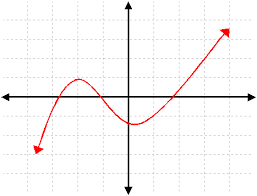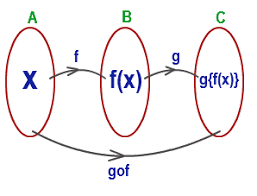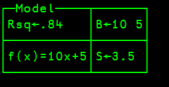# Objects in TamStat

In TamStat, there are four types of objects: data, functions, operators, and namespaces.

Type Description Examples from MathematicsData can be either numeric (quantitative) or character (qualitative).  Data can be structured as a scalar (a single value), vector (a list of values) or matrix (a table of values).
Numeric: 3250.17
Character: 'Joe'
Scalar: a single value, e.g. 2
Vector: a list of values e.g. (3,4,1,7,4)
Matrix: a table of values, e.g.
 2.5 3.1 5.7 5.2 4 2.3

Functions;A function is a set of ordered pairs, sometimes defined by a mathematical rule.

y = f(x)

OperatorsAn operator modifies a function or combines two functions to produce a new function.

 Monadic Operators: Derivative: f'(x) Inverse: f-1(x) Dyadic Operators: Composition f∘g Inner product

NamespacesNamespaces are objects which contain data, functions and operators.

Complex set of results.